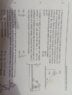# Rotating wedge problem

Prabs3257
Homework Statement:
A wedge is fixed on a moving Square Plate with angular velocity w = √(g/l) and side of the plate is l then the coefficient of friction between the block and the wedge such that there is no relative motion ?? assuming that side of plate is very large as compared to width and height of the wedge
Relevant Equations:
Fc = mv^2/r
I tried making the fbd of both blocks the block on the incline will have mgsin30 friction force, tension and centrepetal force's cos component similarly second block will have mg tension and friction where normal will be centrepetal force after solving my ans is not matching

#### Attachments

•15647749984928646795485040492082.jpg
54.6 KB · Views: 237

Homework Helper
Gold Member
2022 Award
the block on the incline will have mgsin30 friction force,
I assume you mean that will be its normal force, but you are forgetting the rotation.
Please post an fbd for that block.

Prabs3257
I assume you mean that will be its normal force, but you are forgetting the rotation.
Please post an fbd for that block.
for the block on incline i got the quation umg√3/2 + mg/2 = T + mw2l√3/2

and for the other block mg = T + umw2l

by solving these two equation i got the value of u but that's not matching please tell me where i am wrong

Homework Helper
Gold Member
2022 Award
umg√3/2
As I wrote, this is wrong.
What is the equation for the normal force on the block?

Prabs3257
As I wrote, this is wrong.
What is the equation for the normal force on the block?
Is the normal not mgcos30 ??

Prabs3257
Is the normal not mgcos30 ??
Ohhhhh i got it centrifugal force will also affect the normal

Prabs3257
As I wrote, this is wrong.
What is the equation for the normal force on the block?
Thanks i corrected my mistake and got the correct ans